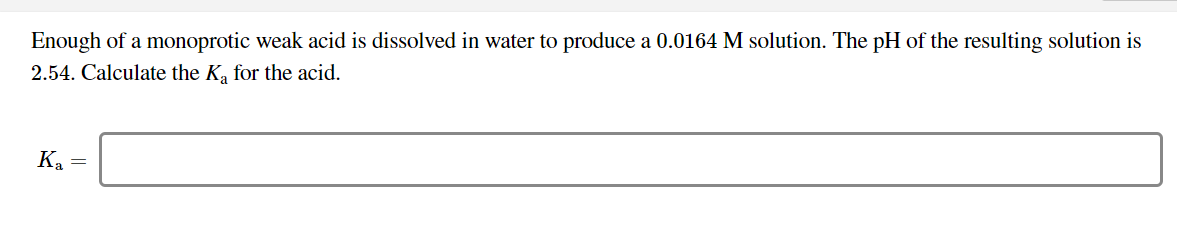# Enough of a monoprotic weak acid is dissolved in water to produce a 0.0164 M solution. The pH of the resulting solution is2.54. Calculate the Ka for the acid.Ka =

Question
19 viewshelp_outlineImage TranscriptioncloseEnough of a monoprotic weak acid is dissolved in water to produce a 0.0164 M solution. The pH of the resulting solution is 2.54. Calculate the Ka for the acid. Ka = fullscreen
check_circle

Step 1

An acid dissociation constant which is denoted by ‘Ka’ can be defined as the quantitative measure of the strength of a given acid in the solution.

Step 2

The pH of a given solution can be defined as the measure of the molar concentration of the hydrogen ions present in the solution and is a measure of its basicity or the acidity. The mathematical formula is as follows:

Step 3

The pH of the resultant solution is given to be 2.54.

The concentration of the resultant solution is giv...

### Want to see the full answer?

See Solution

#### Want to see this answer and more?

Solutions are written by subject experts who are available 24/7. Questions are typically answered within 1 hour.*

See Solution
*Response times may vary by subject and question.
Tagged in

### Physical Chemistry